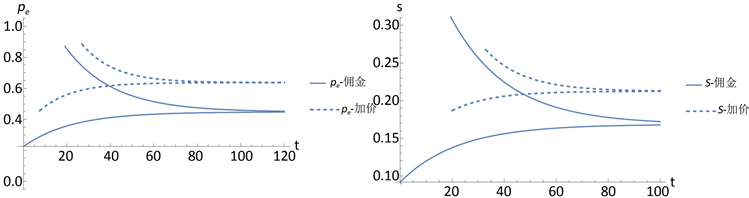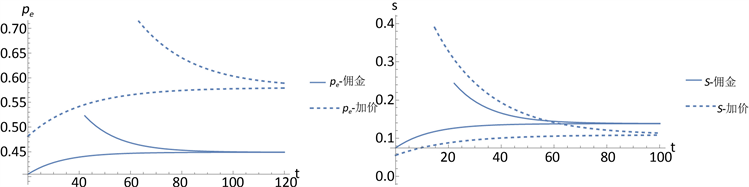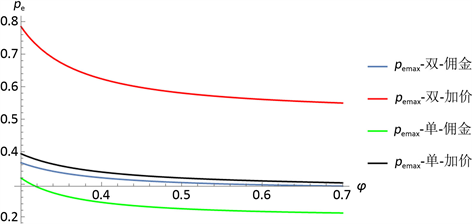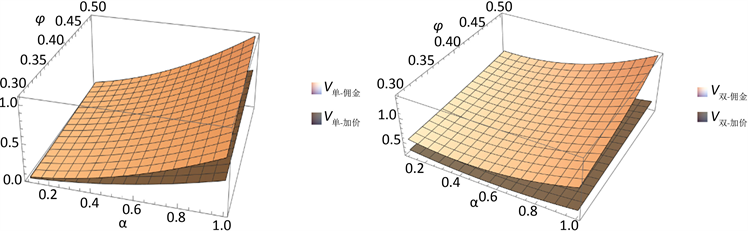#### 期刊菜单

Research on Dynamic Pricing Decision of Brands Considering the Influence of Live Streaming

Abstract: With the development of live broadcast e-commerce, the influence of online celebrity anchors has played an important role in live broadcast e-commerce, attracting the attention of a large number of brands. Whether brands open live broadcast channels and how to give full play to the influence of popular webmasters are important issues for their benefit growth. This paper considers the influence of online celebrity anchors on live broadcast, considers the “price increase” and “commission” models of each channel for single live broadcast channel and dual channels of live broadcast and direct sales, explores the optimal pricing strategies of brands and online celebrity anchors respectively, and analyzes the influence of online celebrity anchors and the influence of the proportion of live broadcast market demand on the balanced decision-making of brands and online celebrity anchors. The research results show that both the live broadcast price of the product and the service effort of the popular webmaster will reach a stable state over time, and depend on the difference between the initial traffic volume of the popular webmaster and the traffic volume of the stable popular webmaster; When online celebrity anchors have great influence, brands are more willing to reduce the price of live product, further stimulate the efforts of online celebrity anchors in live service, so as to achieve the optimal profit. Compared with the single channel, the overall profit of the supply chain under the dual channel of “direct sales + live broadcast” is better, and the online celebrity “commission” mode is better than the online celebrity “price increase” mode. As long as the commission ratio and pit fee between the online celebrity anchor and the brand are set reasonably, both parties can achieve a “double-win”.

1. 引言

2. 国内外研究现状

3. 问题描述及假设

$\text{d}N\left(t\right)=\left[\phi s\left(t\right)-\mu N\left(t\right)\right]\text{d}t$ (1)

$N\left(0\right)=\phi {N}_{0}\ge 0$ (2)

${Q}_{e}=\alpha +N\left(t\right)-{p}_{e}\left(t\right)+m\left({p}_{d}\left(t\right)-{p}_{e}\left(t\right)\right)$ (3)

${Q}_{d}=1-\alpha -{p}_{d}\left(t\right)+m\left({p}_{e}\left(t\right)-{p}_{d}\left(t\right)\right)$ (4)Table 1. Description of parameters and symbols

4. 模型的构建及求解

4.1. 单一渠道模式

4.1.1. 单一线上直销基础模式(策略NT)

$\underset{{p}_{d}\left(t\right)}{\mathrm{max}}{\pi }_{M}^{NT}=E\underset{0}{\overset{\infty }{\int }}{\text{e}}^{-\rho t}\left\{{p}_{d}\left(t\right)\left[1-{p}_{d}\left(t\right)\right]\right\}\text{d}t$ (5)

4.1.2. 单一直播加价模式(策略NY)

$\underset{w\left(t\right)}{\mathrm{max}}{\pi }_{M}^{NY}=E\underset{0}{\overset{\infty }{\int }}{\text{e}}^{-\rho t}\left\{w\left(t\right)\left[\alpha -\left(w\left(t\right)+d\left(t\right)\right)+N\left(t\right)\right]\right\}\text{d}t$ (6)

$\underset{d\left(t\right),s\left(t\right)}{\mathrm{max}}{\pi }_{O}^{NY}=E\underset{0}{\overset{\infty }{\int }}{\text{e}}^{-\rho t}\left\{d\left(t\right)\left[\alpha -\left(w\left(t\right)+d\left(t\right)\right)+N\left(t\right)\right]-\frac{c{s}^{2}\left(t\right)}{2}\right\}\text{d}t$ (7)

$\begin{array}{l}\underset{w}{\mathrm{max}}{\pi }_{M}^{NY}\left(w;s,d\right)\\ \text{s}\text{.t}\left\{\begin{array}{l}\underset{s,d}{\mathrm{max}}{\pi }_{O}^{NY}\left(w;s,d\right)\\ \text{d}N\left(t\right)=\left[\phi s\left(t\right)-\mu N\left(t\right)\right]\text{d}t,N\left(0\right)=\phi {N}_{0}\ge 0\end{array}\end{array}$ (8)

$\left\{\begin{array}{l}{V}^{M*}={a}_{1}^{NY*}{N}^{2}+{a}_{2}^{NY*}N+{a}_{3}^{NY*}\\ {V}^{O*}={b}_{1}^{NY*}{N}^{2}+{b}_{2}^{NY*}N+{b}_{3}^{NY*}\end{array}$ (9)

$\left\{\begin{array}{l}{a}_{1}^{NY*}=\frac{1}{8f}\\ {a}_{2}^{NY*}=\frac{\alpha \left(4cf\left(f+\rho \right)+{\phi }^{2}\right)}{8cf{\left(f+\rho \right)}^{2}}\\ {a}_{3}^{NY*}=\frac{{\alpha }^{2}\left(4{c}^{2}f{\left(f+\rho \right)}^{3}+4cf{\phi }^{2}\left(f+\rho \right)+{\phi }^{4}\right)}{32{c}^{2}f\rho {\left(f+\rho \right)}^{3}}\end{array}$$\left\{\begin{array}{l}{b}_{1}^{NY*}=\frac{c\left(-f-2\mu +\rho \right)}{4{\phi }^{2}}\\ {b}_{2}^{NY*}=\frac{\alpha }{4\left(f+\rho \right)}\\ {b}_{3}^{NY*}=\frac{{\alpha }^{2}\left(2c{\left(f+\rho \right)}^{2}+{\phi }^{2}\right)}{32c\rho {\left(f+\rho \right)}^{2}}\end{array}$

${w}^{NY*}={w}^{NY**}+\frac{1}{2}\left(\phi {N}_{0}-{N}^{**}\right){\text{e}}^{-\frac{1}{2}t\left(\rho -4\mu -f\right)}$ (10)

${d}^{NY*}={d}^{NY**}+\frac{1}{4}\left(\phi {N}_{0}-{N}^{NY**}\right){\text{e}}^{-\frac{1}{2}t\left(\rho -4\mu -f\right)}$ (11)

${s}^{NY*}={s}^{NY**}+\left(\frac{\rho -2\mu -f}{2\phi }\right)\left(\phi {N}_{0}-{N}^{NY**}\right){\text{e}}^{-\frac{1}{2}t\left(\rho -4\mu -f\right)}$ (12)

${p}_{e}^{NY*}={p}_{e}^{NY**}+\frac{3}{4}\left(\phi {N}_{0}-{N}^{NY**}\right){\text{e}}^{-\frac{1}{2}t\left(\rho -4\mu -f\right)}$ (13)

${N}^{NY*}=\left(\phi {N}_{0}-{N}^{NY**}\right){\text{e}}^{-\frac{1}{2}t\left(\rho -4\mu -f\right)}+{N}^{NY**}$ (14)

${N}^{NY**}=\frac{\alpha {\phi }^{2}}{-8c{\mu }^{2}+{\phi }^{2}-8c\mu f}$$f=\sqrt{{\left(2\mu -\rho \right)}^{2}-\frac{{\phi }^{2}}{2c}}$

4.1.3. 单一直播佣金模式(策略NR)

$\underset{{p}_{e}\left(t\right)}{\mathrm{max}}{\pi }_{M}^{NR}=E\underset{0}{\overset{\infty }{\int }}{\text{e}}^{-\rho t}\left\{\left(1-\lambda \right){p}_{e}\left(t\right)\left[\alpha -{p}_{e}\left(t\right)+N\left(t\right)\right]-F\right\}\text{d}t$ (15)

$\underset{s\left(t\right)}{\mathrm{max}}{\pi }_{O}^{NR}=E\underset{0}{\overset{\infty }{\int }}{\text{e}}^{-\rho t}\left\{\lambda {p}_{e}\left(t\right)\left[\alpha -{p}_{e}\left(t\right)+N\left(t\right)\right]-\frac{c{s}^{2}\left(t\right)}{2}+F\right\}\text{d}t$ (16)

$\begin{array}{l}\underset{{p}_{e}}{\mathrm{max}}{\pi }_{M}^{NR}\left({p}_{e};s\right)\\ \text{s}\text{.t}\left\{\begin{array}{l}\underset{s}{\mathrm{max}}{\pi }_{O}^{NR}\left({p}_{e};s\right)\\ \text{d}N\left(t\right)=\left[\phi s\left(t\right)-\mu N\left(t\right)\right]\text{d}t,N\left(0\right)=\phi {N}_{0}\ge 0\end{array}\end{array}$ (17)

$\left\{\begin{array}{l}{V}_{M}^{NR*}={a}_{4}^{NR*}{N}^{2}+{a}_{5}^{NR*}N+{a}_{6}^{NR*}\\ {V}_{O}^{NR*}={b}_{4}^{NR*}{N}^{2}+{b}_{5}^{NR*}N+{b}_{6}^{NR*}\end{array}$ (18)

$\left\{\begin{array}{l}{a}_{4}^{NR*}=-\frac{\lambda -1}{4\left(M+{\rho }^{2}-\rho \right)}\\ {a}_{5}^{NR*}=-\frac{\alpha \left(\lambda -1\right)\left(c\left({\rho }^{2}M+4{\mu }^{2}+4\mu \rho +{\rho }^{3}\right)-\lambda {\phi }^{2}\right)}{c\left(M+\rho \right)\left(M+{\rho }^{2}-\rho \right)\left(M+2{\rho }^{2}-\rho \right)}\\ {a}_{6}^{NR*}=\frac{{\alpha }^{2}\left(-\lambda \right)+{\alpha }^{2}-4F}{4{\rho }^{2}}-\frac{{\alpha }^{2}\left(\lambda -1\right)\lambda {\phi }^{2}\left(c\left({\rho }^{2}M+4{\mu }^{2}+4\mu \rho +{\rho }^{3}\right)-\lambda {\phi }^{2}\right)}{{\rho }^{2}{c}^{2}{\left(M+\rho \right)}^{2}\left(M+{\rho }^{2}-\rho \right)\left(M+2{\rho }^{2}-\rho \right)}\end{array}$ (19)

$\left\{\begin{array}{l}{b}_{4}^{NR*}=\frac{c\left(-M+2\mu +\rho \right)}{4{\phi }^{2}}\\ {b}_{5}^{NR*}=\frac{\alpha \lambda }{M+\rho }\\ {b}_{6}^{NR*}=\frac{{\alpha }^{2}{\lambda }^{2}{\phi }^{2}}{2c\rho {\left(M+\rho \right)}^{2}}+\frac{{\alpha }^{2}\lambda +4F}{4\rho }\end{array}$ (20)

${p}_{e}^{NR*}={p}_{e}^{NR**}+\frac{1}{2}\left(\phi {N}_{0}-{N}^{NR**}\right){\text{e}}^{-\frac{1}{2}t\left(\rho -M\right)}$ (21)

${s}^{NR*}={s}^{NR**}+\left(\frac{2\mu +\rho -M}{2\phi }\right)\left(\phi {N}_{0}-{N}^{NR**}\right){\text{e}}^{-\frac{1}{2}t\left(\rho -M\right)}$ (22)

${N}^{NR*}=\left(\phi {N}_{0}-{N}^{NR**}\right){\text{e}}^{-\frac{1}{2}t\left(\rho -M\right)}+{N}^{**}$ (23)

4.2. 双渠道销售模式

4.2.1. 网红“加价”模式下(策略Y)

$\begin{array}{c}\underset{w\left(t\right),{p}_{d}\left(t\right)}{\mathrm{max}}{\pi }_{M}^{Y}=E\underset{0}{\overset{\infty }{\int }}{\text{e}}^{-\rho t}\left\{w\left(t\right)\left[\alpha -\left(w\left(t\right)+d\left(t\right)\right)+m\left({p}_{d}\left(t\right)-w\left(t\right)-d\left(t\right)\right)+N\left(t\right)\right]\\ \text{\hspace{0.17em}}\text{\hspace{0.17em}}+{p}_{d}\left(t\right)\left[1-\alpha -{p}_{d}\left(t\right)+m\left(w\left(t\right)+d\left(t\right)-{p}_{d}\left(t\right)\right)\right]\right\}\text{d}t\end{array}$ (24)

$\underset{d\left(t\right),s\left(t\right)}{\mathrm{max}}{\pi }_{O}^{Y}=E\underset{0}{\overset{\infty }{\int }}{\text{e}}^{-\rho t}\left\{d\left(t\right)\left[\alpha -\left(w\left(t\right)+d\left(t\right)\right)+m\left({p}_{d}\left(t\right)-w\left(t\right)-d\left(t\right)\right)+N\left(t\right)\right]-\frac{c{s}^{2}\left(t\right)}{2}\right\}\text{d}t$ (25)

$\begin{array}{l}\underset{w,{p}_{d}}{\mathrm{max}}{\pi }_{M}^{Y}\left(w,{p}_{d};s,d\right)\\ \text{s}\text{.t}\left\{\begin{array}{l}\underset{s,d}{\mathrm{max}}{\pi }_{O}^{Y}\left(w,{p}_{d};s,d\right)\\ \text{d}N\left(t\right)=\left[\phi s\left(t\right)-\mu N\left(t\right)\right]\text{d}t,N\left(0\right)=\phi {N}_{0}\ge 0\end{array}\end{array}$ (26)

${s}^{Y*}=\frac{{V}_{O}^{Y*}\text{'}\left(N\right)\phi }{c}=\frac{\left(2{b}_{1}^{Y*}N+{b}_{2}^{Y*}\right)\phi }{c}$ (27)

${d}^{Y*}=\frac{N-w-mw+\alpha +m{p}_{d}}{2\left(1+m\right)}$ (28)

${H}_{O}^{Y}=\rho {V}_{O}^{Y*}=\underset{s,d}{\mathrm{max}}\left\{d\left(\alpha -\left(w+d\right)+m\left({p}_{d}-w-d\right)+N\right)-\frac{c{s}^{2}}{2}+{V}_{O}^{Y*}\text{'}\left(N\right)\left(\phi s-\mu N\right)\right\}$ (29)

${s}^{Y*}=\frac{\phi {V}_{O}^{Y*}\text{'}\left(N\right)}{c}$${d}^{Y*}=\frac{N-w-mw+\alpha +m{p}_{d}}{2\left(1+m\right)}$

${p}_{d}^{Y*}=\frac{m-\alpha +Nm+1}{4m+2}$ (30)

${w}^{Y*}=\frac{N+\alpha +m+Nm}{4m+2}$ (31)

${d}^{Y*}=\frac{N+\alpha }{4+4m}$ (32)

${w}^{Y*}+{d}^{Y*}=\frac{N+m+Nm+\alpha }{2+4m}+\frac{N+\alpha }{4+4m}$ (33)

$\begin{array}{l}{H}_{M}^{Y}=\rho {V}_{M}^{Y*}=\underset{{p}_{d},w}{\mathrm{max}}\left\{w\left[\alpha -\left(w+d\right)+m\left({p}_{d}-w-d\right)+N\right]\\ \text{\hspace{0.17em}}\text{\hspace{0.17em}}\text{\hspace{0.17em}}\text{\hspace{0.17em}}\text{\hspace{0.17em}}\text{\hspace{0.17em}}\text{\hspace{0.17em}}\text{\hspace{0.17em}}\text{\hspace{0.17em}}\text{\hspace{0.17em}}\text{\hspace{0.17em}}\text{\hspace{0.17em}}\text{\hspace{0.17em}}\text{\hspace{0.17em}}\text{\hspace{0.17em}}\text{\hspace{0.17em}}\text{\hspace{0.17em}}\text{\hspace{0.17em}}\text{\hspace{0.17em}}+{p}_{d}\left[1-\alpha -{p}_{d}+m\left(w+d-{p}_{d}\right)\right]+{V}_{M}^{Y*}\text{'}\left(N\right)\left(\phi s-\mu N\right)\right\}\end{array}$ (34)

${p}_{d}^{Y*}=\frac{m-\alpha +Nm+1}{4m+2}$${w}^{Y*}=\frac{N+\alpha +m+Nm}{4m+2}$

$\left\{\begin{array}{l}{V}_{M}^{Y*}={a}_{1}^{Y*}{N}^{2}+{a}_{2}^{Y*}N+{a}_{3}^{Y*}\\ {V}_{O}^{Y*}={b}_{1}^{Y*}{N}^{2}+{b}_{2}^{Y*}N+{b}_{3}^{Y*}\end{array}$ (35)

$\left\{\begin{array}{l}{a}_{1}^{Y*}=-\frac{c\left(1+2m+2{m}^{2}\right)}{8\left(1+m\right)\left(1+2m\right)\left(2c\mu -c\rho +4{\phi }^{2}{b}_{1}\right)}\\ {a}_{2}^{Y*}=-\frac{c\left(2m+2{m}^{2}+\alpha \right)+8\left(1+3m+2{m}^{2}\right){\phi }^{2}{a}_{1}{b}_{2}}{4\left(1+m\right)\left(1+2m\right)\left(c\left(\mu -\rho \right)+2{\phi }^{2}{b}_{1}\right)}\\ {a}_{3}^{Y*}=\frac{c\left(2+2{m}^{2}-4\alpha +3{\alpha }^{2}+2m\left(2-2\alpha +{\alpha }^{2}\right)\right)+8\left(1+3m+2{m}^{2}\right){\phi }^{2}{a}_{2}{b}_{2}}{8c\left(1+m\right)\left(1+2m\right)\rho }\end{array}$ (36)

$\left\{\begin{array}{l}{b}_{1}^{Y*}=\frac{-c\left(2\mu -\rho +\sqrt{{\left(\rho -2\mu \right)}^{2}-\frac{{\phi }^{2}}{2c\left(1+m\right)}}\right)}{4{\phi }^{2}}\\ {b}_{2}^{Y*}=-\frac{c\alpha }{8\left(1+m\right)\left(c\mu -c\rho +2{\phi }^{2}{b}_{1}\right)}\\ {b}_{3}^{Y*}=\frac{{b}_{2}^{2}{\phi }^{2}}{2c\rho }+\frac{{\alpha }^{2}}{16\rho \left(1+m\right)}\end{array}$ (37)

$\begin{array}{l}\rho \left({a}_{1}{N}^{2}+{a}_{2}N+{a}_{3}\right)\\ \equiv \frac{{N}^{2}\left(c\left(1+2m+2{m}^{2}\right)+16c\left(1+3m+2{m}^{2}\right)\mu {a}_{1}+32\left(1+3m+2{m}^{2}\right){\phi }^{2}{a}_{1}{b}_{1}\right)}{8c\left(1+m\right)\left(1+2m\right)}\\ \text{\hspace{0.17em}}\text{\hspace{0.17em}}+\frac{N\left(2c\left(2m+2{m}^{2}+\alpha \right)+8c\left(1+3m+2{m}^{2}\right)\mu {a}_{2}+16\left(1+3m+2{m}^{2}\right){\phi }^{2}{a}_{2}{b}_{1}+16\left(1+3m+2{m}^{2}\right){\phi }^{2}{a}_{1}{b}_{2}\right)}{8c\left(1+m\right)\left(1+2m\right)}\\ \text{\hspace{0.17em}}\text{\hspace{0.17em}}+\frac{2c+2c{m}^{2}-4c\alpha +3c{\alpha }^{2}+2cm\left(2-2\alpha +{\alpha }^{2}\right)+8\left(1+3m+2{m}^{2}\right){\phi }^{2}{a}_{2}{b}_{2}}{8c\left(1+m\right)\left(1+2m\right)}\\ \rho \left({b}_{1}{N}^{2}+{b}_{2}N+{b}_{3}\right)\equiv \frac{{\alpha }^{2}}{16\left(1+m\right)}+{N}^{2}\left(\frac{1}{16\left(1+m\right)}+2\mu {b}_{1}+\frac{2{\phi }^{2}{b}_{1}^{2}}{c}\right)+\frac{{\phi }^{2}{b}_{2}^{2}}{2c}+N\left(\frac{\alpha }{8\left(1+m\right)}+\mu {b}_{2}+\frac{2{\phi }^{2}{b}_{1}{b}_{2}}{c}\right)\end{array}$

$\begin{array}{l}{V}_{M}^{Y*}={a}_{1}{N}^{2}+{a}_{2}N+{a}_{3}\\ {V}_{O}^{Y*}={b}_{1}{N}^{2}+{b}_{2}N+{b}_{3}\end{array}$

${w}^{Y*}={w}^{Y**}+\left(\frac{1+m}{4m+2}\right)\left(\phi {N}_{0}-{N}^{Y**}\right){\text{e}}^{-\left(\frac{2*{b}_{4}^{Y*}-c*\mu }{c}\right)t}$ (38)

${d}^{Y*}={d}^{Y**}+\left(\frac{1}{2m+2}\right)\left(\phi {N}_{0}-{N}_{m}^{Y**}\right){\text{e}}^{-\left(\frac{2*{b}_{4}^{Y*}-c*\mu }{c}\right)t}$ (39)

${p}_{d}^{Y*}={p}_{d}^{Y**}+\left(\frac{1+m}{4m+2}\right)\left(\phi {N}_{0}-{N}^{Y**}\right){\text{e}}^{-\left(\frac{2*{b}_{4}^{Y*}-c*\mu }{c}\right)t}$ (40)

${s}^{Y*}={s}^{Y**}+\left(\frac{2\mu +\rho -\rho A}{2\phi }\right)\left(\phi {N}_{0}-{N}^{Y**}\right){\text{e}}^{-\left(\frac{2*{b}_{4}^{Y*}-c*\mu }{c}\right)t}$ (41)

${w}^{Y**}=\frac{m+\alpha }{2+4m}-\frac{2\alpha {\phi }^{2}}{c\left(2+4m\right)\left(8\mu -2\rho +X\right)\left(2\rho +X\right)}$

${d}^{Y**}=\frac{\alpha \left(-{\phi }^{2}+2c\left(1+m\right)\mu \left(2\mu +X\right)\right)}{c{\left(1+m\right)}^{2}\left(8\mu -2\rho +X\right)\left(2\rho +X\right)}$${s}^{Y**}=\frac{\alpha \phi \left(6\mu -2\rho +X\right)}{c\left(1+m\right)\left(8\mu -2\rho +X\right)\left(2\rho +X\right)}$

$X=\sqrt{4{\left(-2\mu +\rho \right)}^{2}-\frac{2{\phi }^{2}}{c+cm}}$

${N}_{Y}^{\text{*}*}=\left(\phi {N}_{0}-\frac{{\phi }^{2}{b}_{2}^{Y*}}{2{b}_{1}^{Y*}{\phi }^{2}-c\mu }\right){\text{e}}^{-\left(\frac{2{b}_{1}^{Y*}{\phi }^{2}-c\mu }{c}\right)t}+\frac{{\phi }^{2}{b}_{2}^{Y*}}{2{b}_{1}^{Y*}{\phi }^{2}-c\mu }$ (42)

$\frac{\partial {N}^{Y**}}{\partial \alpha }<0$$\frac{\partial {w}^{Y**}}{\partial \alpha }>0$$\frac{\partial {d}^{Y**}}{\partial \alpha }>0$$\frac{\partial {s}^{Y**}}{\partial \alpha }>0$$\frac{\partial {p}_{d}^{Y**}}{\partial \alpha }>0$ (43)

$\frac{\partial {p}_{d}^{Y**}}{\partial \phi }<0$$\frac{\partial {w}^{Y**}}{\partial \phi }<0$$\frac{\partial {d}^{Y**}}{\partial \phi }<0$$\frac{\partial {N}^{Y**}}{\partial \phi }>0$ (44)

$\frac{\partial {p}_{d}^{Y**}}{\partial c}>0$$\frac{\partial {w}^{Y**}}{\partial c}>0$$\frac{\partial {d}^{Y**}}{\partial c}>0$$\frac{\partial {N}^{Y**}}{\partial c}<0$ (45)

4.2.2. 佣金模式下(策略R)

$\begin{array}{c}\underset{{p}_{e}\left(t\right),{p}_{d}\left(t\right)}{\mathrm{max}}{\pi }_{M}^{R}=E\underset{0}{\overset{\infty }{\int }}{\text{e}}^{-rt}\left\{\left(1-\lambda \right){p}_{e}\left(t\right)\left[\alpha -{p}_{e}\left(t\right)+m\left({p}_{d}\left(t\right)-{p}_{e}\left(t\right)\right)+N\left(t\right)\right]\\ \text{\hspace{0.17em}}\text{\hspace{0.17em}}+{p}_{d}\left(t\right)\left[1-\alpha -{p}_{d}\left(t\right)+m\left({p}_{e}\left(t\right)-{p}_{d}\left(t\right)\right)\right]-F\right\}\text{d}t\end{array}$ (46)

$\underset{s\left(t\right)}{\mathrm{max}}{\pi }_{O}^{R}=E\underset{0}{\overset{\infty }{\int }}{\text{e}}^{-rt}\left\{\lambda {p}_{e}\left(t\right)\left[\alpha -{p}_{e}\left(t\right)+m\left({p}_{d}\left(t\right)-{p}_{e}\left(t\right)\right)+N\left(t\right)\right]-\frac{c{s}^{2}\left(t\right)}{2}+F\right\}\text{d}t$ (47)

$\begin{array}{l}\underset{{p}_{e},{p}_{d}}{\mathrm{max}}{\pi }_{M}^{R}\left({p}_{e},{p}_{d};s\right)\\ \text{s}\text{.t}\left\{\begin{array}{l}\underset{s}{\mathrm{max}}{\pi }_{O}^{R}\left({p}_{e},{p}_{d};s\right)\\ \text{d}N\left(t\right)=\left[\phi s\left(t\right)-\mu N\left(t\right)\right]\text{d}t,N\left(0\right)=\phi {N}_{0}\ge 0\end{array}\end{array}$ (48)

${s}^{R*}=\frac{\phi {V}_{O}^{R*}\text{'}\left(N\right)}{c}$ (49)

${p}_{d}^{R*}=-\frac{\left(\lambda -1\right)\left(2\left(\alpha -1\right)+m\left(\alpha \lambda +N\left(\lambda -2\right)-2\right)\right)}{4\left(\lambda -1\right)+{\lambda }^{2}{m}^{2}+8\left(\lambda -1\right)m}$ (50)

${p}_{e}^{R*}=\frac{2\left(\lambda -1\right)\left(\alpha +N\right)+m\left(\alpha \lambda +2N\left(\lambda -1\right)+\lambda -2\right)}{4\left(\lambda -1\right)+{\lambda }^{2}{m}^{2}+8\left(\lambda -1\right)m}$ (51)

$\left\{\begin{array}{l}{V}_{M}^{R*}={a}_{4}^{R*}{N}^{2}+{a}_{5}^{R*}N+{a}_{6}^{R*}\\ {V}_{O}^{R*}={b}_{4}^{R*}{N}^{2}+{b}_{5}^{R*}N+{b}_{6}^{R*}\end{array}$ (52)

$\left\{\begin{array}{l}{a}_{4}^{R*}=-\frac{c\left(1+m\right){\left(-1+\lambda \right)}^{2}}{\left(-4-8m+4\lambda +8m\lambda +{m}^{2}{\lambda }^{2}\right)\left(2c\mu +c\rho -4{\phi }^{2}{b}_{4}\right)}\\ {a}_{5}^{R*}=\frac{-\frac{\left(-1+\lambda \right)\left(2\alpha \left(-1+\lambda \right)+m\left(-2+\lambda +\alpha \lambda \right)\right)}{\left(4\left(-1+\lambda \right)+8m\left(-1+\lambda \right)+{m}^{2}{\lambda }^{2}\right)\rho }+\frac{2{\phi }^{2}{a}_{4}{b}_{5}}{c\rho }}{1+\frac{c\mu -2{\phi }^{2}{b}_{4}}{c\rho }}\\ {a}_{6}^{R*}=-\frac{F+\frac{\left(-1+\lambda \right)\left(-1+2\alpha +{\alpha }^{2}\left(-2+\lambda \right)+m\left(-1+\alpha \lambda \right)\right)}{4\left(-1+\lambda \right)+8m\left(-1+\lambda \right)+{m}^{2}{\lambda }^{2}}-\frac{{\phi }^{2}{a}_{5}{b}_{5}}{c}}{\rho }\end{array}$

$\left\{\begin{array}{l}{b}_{4}^{R*}=\frac{c\left(2\mu +\rho \left(\rho -A\right)\right)}{4{\phi }^{2}}\\ {b}_{5}^{R*}=\frac{c\lambda L}{{B}^{2}\left(c\rho +\mu \rho +2{b}_{4}{\phi }^{2}\right)}\\ {b}_{6}^{R*}=\frac{F}{\rho }+\frac{{b}_{5}{}^{2}{\phi }^{2}}{2c\rho }+\frac{\lambda \left(\lambda m\left(1+m\right)+\alpha \left(-2+2\lambda -4m+3\lambda m\right)\right)\left(\left(-2+\lambda \right)m+\alpha \left(-2+\lambda \left(2+m\right)\right)\right)}{{B}^{2}\rho }\end{array}$

$\begin{array}{l}{p}_{e}^{R*}={p}_{e}^{R**}+\left(\frac{-2-2m+2\lambda +2m\lambda }{4\left(-1+\lambda \right)+8m\left(-1+\lambda \right)+{m}^{2}{\lambda }^{2}}\right)\left(\phi {N}_{0}-{N}^{R**}\right){\text{e}}^{-\left(\frac{2\mu +{\rho }^{2}-\rho A}{2{\phi }^{2}}-\mu \right)t}\\ {p}_{d}^{R*}={p}_{d}^{R**}+\left(-\frac{\left(-1+\lambda \right)\left(-2m+m\lambda \right)}{4\left(-1+\lambda \right)+8m\left(-1+\lambda \right)+{m}^{2}{\lambda }^{2}}\right)\left(\phi {N}_{0}-{N}^{R**}\right){\text{e}}^{-\left(\frac{2\mu +{\rho }^{2}-\rho A}{2{\phi }^{2}}-\mu \right)t}\\ {s}^{R*}={s}^{R**}+\left(\frac{2\mu +\rho -\rho A}{2\phi }\right)\left(\phi {N}_{0}-{N}^{R**}\right){\text{e}}^{-\left(\frac{2\mu +{\rho }^{2}-\rho A}{2{\phi }^{2}}-\mu \right)t}\\ {N}_{Y}^{R*}=\left(\phi {N}_{0}-{N}^{R**}\right){\text{e}}^{-\left(\frac{2\mu +{\rho }^{2}-\rho A}{2{\phi }^{2}}-\mu \right)t}+\frac{{b}_{5}^{R*}{\phi }^{2}}{2{\phi }^{2}{b}_{4}^{R*}-c\mu }\end{array}$ (53)

${p}_{d}^{R**}=\frac{\left(-1+\lambda \right)\left(2+2m-2\alpha -m\alpha \lambda \right)}{B}+\frac{4m\left(-2+\lambda \right)\left(-1+\lambda \right)\lambda L{\phi }^{2}}{c{B}^{3}{\rho }^{2}\left({A}^{2}-1\right)}$${N}^{R**}=\frac{{b}_{5}^{*}{\phi }^{2}}{2{\phi }^{2}{b}_{4}^{*}-c\mu }$

${p}_{e}^{R**}=\frac{2\alpha \left(-1+\lambda \right)+m\left(-2+\lambda +\alpha \lambda \right)}{4\left(-1+\lambda \right)+8m\left(-1+\lambda \right)+{m}^{2}{\lambda }^{2}}-\frac{8\left(1+m\right)\left(-1+\lambda \right)\lambda L{\phi }^{2}}{c{B}^{3}{\rho }^{2}\left({A}^{2}-1\right)}$${s}_{}^{R**}=-\frac{4\lambda L\left(\mu +\rho -\rho A\right)}{c{B}^{2}{\rho }^{2}\left({A}^{2}-1\right)}$

$A=\frac{1}{\rho }\sqrt{{\left(2\mu +\rho \right)}^{2}-\frac{16\left(1+m\right)\left(-1+\lambda \right)\lambda \left(2\left(-1+\lambda \right)+4m\left(-1+\lambda \right)+{m}^{2}\lambda \right){\phi }^{2}}{c{B}^{2}}}$

$B=4\left(-1+\lambda \right)+8m\left(-1+\lambda \right)+{m}^{2}{\lambda }^{2}$

$L=8\alpha {\left(-1+\lambda \right)}^{2}+4m\left(1+5\alpha \right){\left(-1+\lambda \right)}^{2}+4{m}^{2}\left(-1+\lambda \right)\left(-2-2\alpha +2\lambda +3\alpha \lambda \right)+{m}^{3}\lambda \left(-4+\left(3+\alpha \right)\lambda \right)>0$

$\frac{\partial {p}_{e}^{R**}}{\partial \alpha }\left\{\begin{array}{ll}>0\hfill & \lambda \in \left[0,\frac{2}{2+m}\right]\hfill \\ <0\hfill & \lambda \in \left[\frac{2}{2+m},\frac{2+4m}{2+4m+{m}^{2}}\right]\hfill \end{array}$$\frac{\partial {p}_{d}^{R**}}{\partial \alpha }<0$$\frac{\partial {s}^{R**}}{\partial \alpha }>0$$\frac{\partial {N}^{R**}}{\partial \alpha }<0$ (54)

1) 当 $\lambda \in \left[0,\frac{2}{2+m}\right]$ 时，产品的直播价格随着直播渠道需求比例的扩大而提高；当 $\lambda \in \left[\frac{2}{2+m},\frac{2+4m}{2+4m+{m}^{2}}\right]$ 时，产品的直播价格随着直播渠道需求比例的扩大而降低。

2) 品牌商的直销价格总是随着直播渠道市场规模比例的扩大而降低，也就是说，品牌商总是希望通过降低价格来获利。

3) 网红主播的直播服务水平随着直播渠道市场规模比例的扩大而提高。

$\frac{\partial {p}_{e}^{R**}}{\partial c}>0$$\frac{\partial {p}_{d}^{R**}}{\partial c}>0$$\frac{\partial {s}^{R**}}{\partial c}<0$$\frac{\partial {N}^{R**}}{\partial c}<0$ (55)

5. 数值分析

5.1. 产品直播价格和网红主播服务努力程度的最优路径分析(a) (b)(c) (d)Figure1. The path map of the best live product price under Single Channel/Dual Channels图1. 单/双渠道下最优产品直播价格和直播服务水平路径图

5.2. 网红主播影响力对产品直播价格的影响分析网红主播可通过自身的影响力，扩大产品的市场需求，起到一定的引流效果，从而会对品牌商和网红主播的价格和服务努力决策产生影响。图2刻画了不同渠道策略下网红主播的影响力对产品的直播价格的影响。从图2可知，无论何种策略下，产品的直播价格均随着网红主播影响力的提高而降低，相对加价模式，佣金策略下产品的直播价格较低。这是由于网红主播的影响力较大意味着引流量的初始值也将处于高位，对产品的需求影响较高，品牌商也愿意为提高产品需求而降低价格。这也可以说明在现实中，为什么影响力较大的网红主播具有一定的议价能力，可以与品牌产品谈到一个较低的价格。Figure 2. The influence of anchor influence on the price of live product r

5.3. 最优利润值分析(a) (b)

Figure 3. The Influence of α and m on the Profit of Supply Chain under Single/Dual Channels

6. 结论与展望

1) 当品牌商引入网红直播渠道时，无论采取何种模式，网红主播的直播服务努力程度均随时间而单调变化，且取决于网红主播的初始引流量以及稳态网红主播引流量之间的差距。同时，品牌商和网红主播的均衡动态定价策略也受到网红初始引流量和稳态引流量的影响。当网红主播的影响力较大，初始引流量大于稳态均衡引流量时，网红主播的直播服务努力程度随着引流量的增加而降低，品牌商和网红主播采取价格撇除策略，即随着时间的推移，降低产品的批发价和网红加价价格；反之亦反。

2) 无论何种渠道下，产品的直播价格与网红主播的影响力成反比，网红主播的直播服务努力与网红主播的影响力成正比。当网红影响力越大时，网红主播的直播引流量越大，产品的直播需求量越大，网红的议价能力也将会有所体现，故产品的直播价格会随之降低。另外，当网红主播的影响力越大，其单位直播服务努力所带来的边际效益越大，加之产品直播价格的降低，均激励网红主播提高其直播服务努力程度。此外，采取网红“佣金”模式时的产品直播价格均会低于采取网红“加价”模式下的产品直播价格，而其直播服务努力程度也较高。

3) 直播市场需求比例会对品牌商和网红主播的策略产生影响。当直播市场需求比例较大时，意味着直播市场具有较大潜力，网红主播更愿意提高直播服务努力程度；品牌商会随着直播需求比例的提高而降低产品的直销价格，提高产品的直播价格。该结论表明，在直播市场需求比例较高时，网红主播的直播服务努力程度会被激励，品牌商更倾向于直播渠道中提高直播价格，直销渠道中降低直销价格来获取更大的利润。

4) 网红“佣金”模式下供应链整体的利润要高于网红“加价”模式下的供应链整体利润。随着直播市场需求比例的扩大，双渠道下供应链整体利润先减少后增大。直播市场需求比例过小，其低价策略会使得整体利润下降，当直播市场比例较大时，产品的直播将有所提高，网红主播的引流优势也将会突显，从而供应链整体利润上升。这也侧面说明在刚刚开通直播渠道时，直播市场比例较小，供应链整体利润是呈现下降趋势，但是一旦打通直播渠道，具备一定的“粉丝”基础，供应链的整体利润将会有所提高，这也是为什么许多直销店铺要开通直播间的原因。现实中许多网红店铺就是先吸引粉丝，具备一定流量后，直播卖货并取得了较好的成效。只要能够合理的制定分配比例和坑位费，品牌商和网红主播便能实现“双赢”的局面。

  刘凤军, 孟陆, 陈斯允, 段珅. 网红直播对消费者购买意愿的影响及其机制研究[J]. 管理学报, 2020, 17(1): 94-104.  梁玲, 袁璐华, 谢家平. 基于ABC态度理论的直播带货用户购买行为机理实证[J]. 软科学, 2022, 19(5): 1-12.  Wang, X., et al. (2019) An Analysis of Salary Mechanisms in the Sharing Economy: The Interaction between Streamers and Unions. International Journal of Production Economics, 214, 106-124. https://doi.org/10.1016/j.ijpe.2019.04.003  郑森圭, 苏丹华, 汪寿阳, 尚维. 直播平台打赏收入分成模式研究[J]. 系统工程理论与实践, 2020, 40(5): 1221-1228.  邢鹏, 尤浩宇, 樊玉臣. 考虑平台营销努力的直播电商服务供应链质量努力策略[J]. 控制与决策, 2022, 37(1): 205-212.  郭延禄, 罗公利, 侯贵生, 王晓彤. “种草”与“翻车”: 网红直播带货的产品质量问题与治理研究[J]. 中国管理科学, 2021, 26(5): 1-15.  于天阳, 关志民, 董经洋, 曲优. 不同权力结构下考虑网红营销努力的直播电商供应链决策研究[J]. 管理学报, 2022, 19(5): 714-722+748.  鲁馨蔓, 李艳霞, 王君, 余思勤. 云服务供应链技术创新与动态定价的微分博弈分析[J]. 运筹与管理, 2020, 29(6): 49-57.  段永瑞, 徐建, 霍佳震. 考虑参照效应的自有品牌动态定价策略[J]. 工业工程与管理, 2017, 22(1): 14-21.  徐琪, 杨玉莹. 基于双边平台匹配努力的共享供应链动态定价策略研究[J]. 运筹与管理, 2022, 31(6): 82-90.  叶欣, 周艳菊. 考虑商誉的双渠道供应链动态定价与联合减排策略[J]. 中国管理科学, 2021, 29(2): 117-128.  胡娇, 李莉, 张华, 朱星圳, 杨文胜. 考虑参照效应和主播影响力的网络直播平台动态定价决策[J]. 系统工程理论与实践, 2022, 42(3): 755-766.  Lu, F.X., Zhang, J.X. and Tang, W.S. (2019) Wholesale Price Contract versus Consignment Contract in a Supply Chain Considering Dynamic Advertising. International Transactions in Operational Research, 26, No. 5.